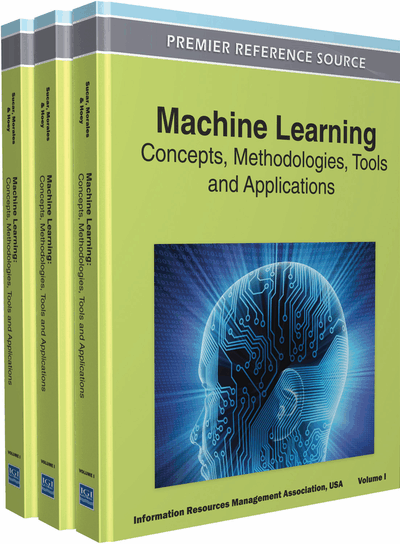# Calibration of Machine Learning Models

Antonio Bella, Cèsar Ferri, José Hernández-Orallo, María José Ramírez-Quintana
DOI: 10.4018/978-1-60960-818-7.ch104
OnDemand:
(Individual Chapters)
Available
\$37.50
No Current Special Offers

## Abstract

The evaluation of machine learning models is a crucial step before their application because it is essential to assess how well a model will behave for every single case. In many real applications, not only is it important to know the “total” or the “average” error of the model, it is also important to know how this error is distributed and how well confidence or probability estimations are made. Many current machine learning techniques are good in overall results but have a bad distribution assessment of the error. For these cases, calibration techniques have been developed as postprocessing techniques in order to improve the probability estimation or the error distribution of an existing model. This chapter presents the most common calibration techniques and calibration measures. Both classification and regression are covered, and a taxonomy of calibration techniques is established. Special attention is given to probabilistic classifier calibration.
Chapter Preview
Top

## Introduction

One of the main goals of machine learning methods is to build a model or hypothesis from a set of data (also called evidence). After this learning process, the quality of the hypothesis must be evaluated as precisely as possible. For instance, if prediction errors have negative consequences in a certain application domain of a model (for example, detection of carcinogenic cells), it is important to know the exact accuracy of the model. Therefore, the model evaluation stage is crucial for the real application of machine learning techniques. Generally, the quality of predictive models is evaluated by using a training set and a test set (which are usually obtained by partitioning the evidence into two disjoint sets) or by using some kind of cross-validation or bootstrap if more reliable estimations are desired. These evaluation methods work for any kind of estimation measure. It is important to note that different measures can be used depending on the model. For classification models, the most common measures are accuracy (the inverse of error), f-measure, or macro-average. In probabilistic classification, besides the percentage of correctly classified instances, other measures such as logloss, mean squared error (MSE) (or Brier’s score) or area under the ROC curve (AUC) are used. For regression models, the most common measures are MSE, the mean absolute error (MAE), or the correlation coefficient.

With the same result for a quality metric (e.g. MAE), two different models might have a different error distribution. For instance, a regression model R1 that always predicts the true value plus 1 has a MAE of 1. However, it is different to a model R2 that predicts the true value for n - 1 examples and has an error of n for one example. Model R1 seems to be more reliable or stable, i.e., its error is more predictable. Similarly, two different models might have a different error assessment with the same result for a quality metric (e.g. accuracy). For instance, a classification model C1 which is correct 90% of the cases with a confidence of 0.91 for every prediction is preferable to model C2 which is correct 90% of the cases with a confidence of 0.99 for every prediction. The error self-assessment, i.e., the purported confidence, is more accurate in C1 than in C2.

In both cases (classification and regression), an overall picture of the empirical results is helpful in order to improve the reliability or confidence of the models. In the case of regression, the model R1, which always predicts the true value plus 1, is clearly uncalibrated, since predictions are usually 1 unit above the real value. By subtracting 1 unit from all the predictions, R1 could be calibrated and interestingly, R2 can be calibrated in the same way. In the case of classification, a global calibration requires the confidence estimation to be around 0.9 since the models are right 90% of the time.

Thus, calibration can be understood in many ways, but it is usually built around two related issues: how error is distributed and how self-assessment (confidence or probability estimation) is performed. Even though both ideas can be applied to both regression and classification, this chapter focuses on error distribution for regression and self-assessment for classification.

## Complete Chapter List

Search this Book:
Reset